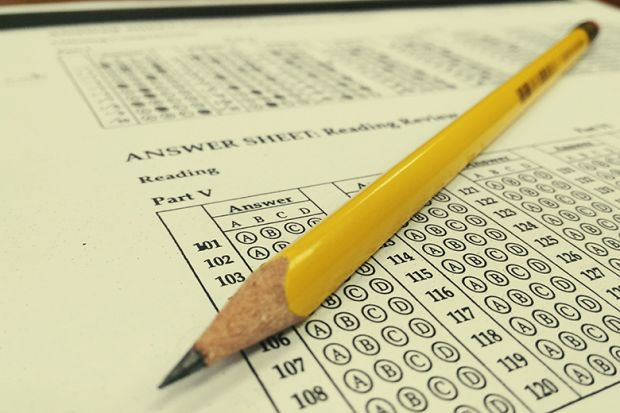September 27, 2023The branch of mathematics that deals with the collection, organization, analysis, and interpretation of data is called statistics. It uses quantified models, representations, and synopses for a given set of experimental data or real-life studies. It also studies methodologies to gather, review, analyze, and draw conclusions from data.

Statistics is an important topic for the JEE Main exam. Two important statistical measures are mean and variance. It is a measure of central dispersion. Central dispersion refers to how the data that we are taking for observation are scattered and distributed. Mean refers to the average of a given set of observations. The average of the squared difference from the mean gives the variance.

## Mean

The average of a random variable in probability and statistics is the mean or the expected value. If the probability distribution for a random variable is known, we can also find its expected value. The mean shows the position or the central tendency of the random variable. Its definition differs in different branches of mathematics. Normally, we denote the average of the discrete data present in a set of numbers. For ungrouped data, the arithmetic mean is given by

Here n denotes the number of terms and x1, x2, x3,… denotes the respective observations.

## Properties of Mean

• If individual units are increased by k, then the mean will increase by k.
• If individual units are decreased by k, then the mean will decrease by k.
• If each unit is multiplied by k, then the mean will be multiplied by k.
• If each unit is divided by k, then the mean will be divided by k.

## Variance

It is the average of the squared differences from the mean. It calculates how far a data set is spread out. Sometimes we take the mean deviation by taking the absolute values from a set of values. The absolute values are taken to measure the deviations, else, the positive and negative deviation may cancel out each other.

In order, to remove the sign of deviation, we take the variance of the data set, i.e. we square the deviation values. Since the squares are always positive, the variance is always a positive number. Let us see the steps to find it.

### Steps to find the Variance

1. Calculate the mean.

2. Subtract the mean from each number.

3. Square the difference.

4. Find the average of the squared differences.

The variance is denoted by

### JEE Statistics Previous Year Questions

As far as the JEE Main exam is concerned, statistics is an important topic. Students are recommended to learn JEE Statistics previous year questions with solutions so that they can get an idea on question pattern and marking scheme. These solutions will help students to understand the difficulty level of questions asked for the JEE Main exam. Students can easily access these solutions and download them in PDF format for free.

All the step by step solutions are prepared by specialized experts. Practising previous year question papers help students to improve their speed and accuracy. This helps students to be more confident during the exam.